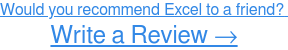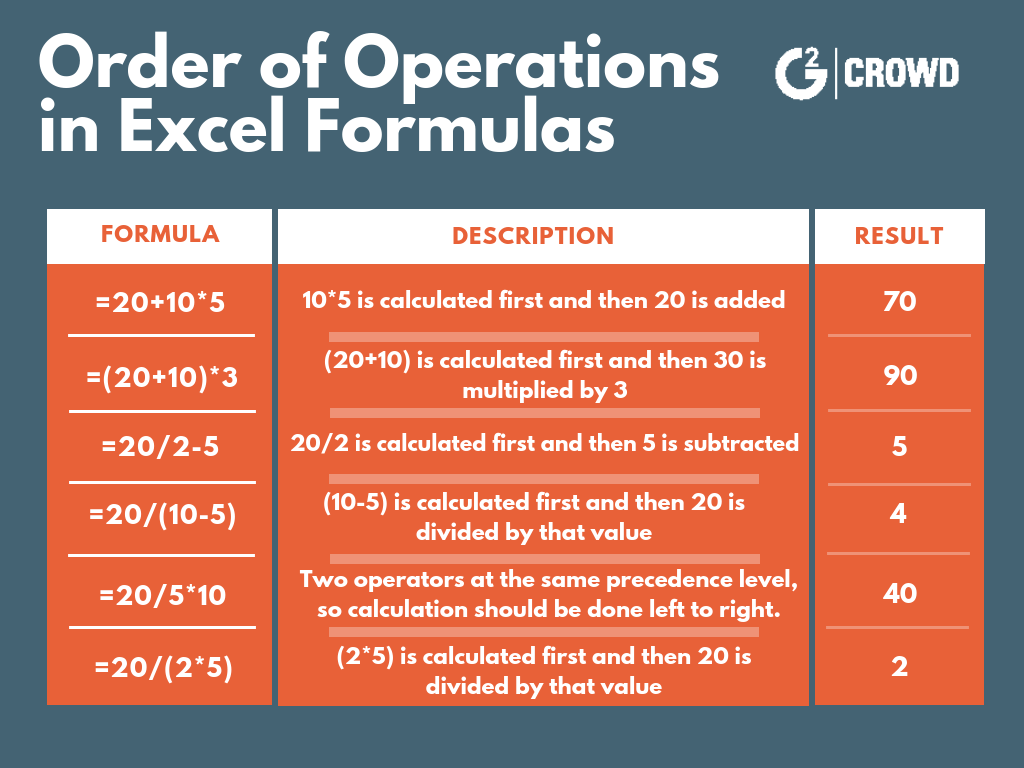# How to Subtract in Excel in 2019 (+ Examples and Screenshots)

So you're here to learn how to subtract in Microsoft Excel. No problem!

In order to learn how to subtract in Excel, you'll need to know how to write Excel formulas.

That being said, I'm here to walk you through it, and I'll provide examples and screenshots along the way.

Here's everything you need to know about subtracting in Excel:

Related: Want to know more about Microsoft Excel? Learn how to add in Excelhow to multiply in Excel and how to divide in Excel!

# How to subtract in Excel

If you’re looking to subtract in Excel, you’ll need to know what formulas are and how to write them.

Excel formulas are expressions used in Excel to display values that are calculated from the data you’ve entered and they’re generally used to perform various mathematical, statistical, and logical operations.So before we jump into the specifics of how to subtract in Excel, here are a few reminders to keep in mind when writing Excel formulas:

• All Excel formulas should begin with the equal sign (=). This is a must in order for Excel to recognize it as a formula.
• The cells will display the result of the formula, not the actual formula. So by using cell references rather than just entering the data in the cell, if you need to go back later the values will be easier to understand.
• When using arithmetic operators in your formula, remember the order of operations (I personally reference the acronym PEMDAS: Parenthesis, Exponentiation, Multiplication, Division, Addition, Subtraction.) Below you’ll find an example of how the order of operations is applied to formulas in Excel.## How to subtract with numbers in a cell

To subtract numbers in a cell, you'll need to write your formula in the designated cell. Your formula should start with the equal sign (=), and contain the arithmetic operator needed for the calculation. In this case, you want to subtract 5 from 15, so your formula is =15-5.Once you’ve entered the formula, press ‘Enter’ and your result will populate. In this example, the subtraction formula of =15-5 yields a result of 10 (see below).## How to subtract with cell references in a cell

In this case, your data will need to be in its own cells.

You can see below that a subtracting formula was written in cell B2 using cell references, but the data had to be entered separately in cells A2 and A3.## How to subtract numbers in a range

Subtracting numbers in a range will be a little different. Because there is no SUBTRACT function in Excel, you’ll use the SUM function to subtract numbers in a range.

To do this, you’ll have to convert any numbers that you want to subtract to their negative values (because adding a negative number is the same as subtracting). Enter each of these values in separate cells giving them their own cell reference.

Once you’ve entered the data and converted the values, use the SUM function (you can find this under 'Insert Function' or start typing =Sum in the cell) and enter the range of the values you want to subtract.

Do this by entering the cell reference of the first cell in the range (A2), followed by colon (:) and the cell reference of the last cell in the range (A4).After you have the function and cell range entered, press ‘Enter’ and your result will populate!

In this case, 10 and 5 were subtracted from 25, resulting in 10.## You’re ready to subtract in Excel

Now grab your computer and dive into Excel to give it a try for yourself!

Want to learn more about Excel? Learn how to lock cells in Excel and how to make a line graph in Excel!

Be sure to stay tuned for more articles soon!

#### Jordan Wahl

Jordan Wahl is a senior content marketing and SEO specialist who joined G2 after graduating with her BBA in Marketing from the University of Wisconsin-Whitewater. She loves anything that puts her in her creative space – including writing, art, and music (her absolute favorite).

Technology
Sales
Technology